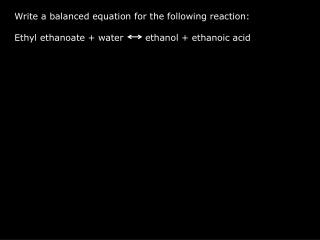DownloadDownload PresentationWrite a balanced equation for the following reaction:

# Write a balanced equation for the following reaction:

Download Presentation## Write a balanced equation for the following reaction:

- - - - - - - - - - - - - - - - - - - - - - - - - - - E N D - - - - - - - - - - - - - - - - - - - - - - - - - - -
##### Presentation Transcript

1. Write a balanced equation for the following reaction: Ethyl ethanoate + water ethanol + ethanoic acid

2. 1a 1b 2 3 4 1) Mass of empty tube / g 12.01 12.24 12.57 12.18 12.73 2) Add 2M HCl catalyst / cm3 5.0 5.0 5.0 5.0 5.0 3) New mass / g 17.03 17.34 17.77 17.35 17.92 4) Add ethyl ethanoate / cm3 0 0 5.0 4.0 2.0 5) New mass / g 22.28 20.99 19.78 6) Add water / cm3 1.0 3.0 7) New mass / g 21.98 22.78

3. Leave for 48 hours to reach equilibrium (shake occasionally during this time)

4. Fill a burette with 1M NaOH solution Titate the contents of each of the 5 tubes For each tube, make sure all the contents have been emptied into the conical flask by flushing the tube out with distilled water. Use phenolthalein indicator solution. Table showing tube number and titre in cm3

5. Write an expression for Kc for this reaction. Aim - Work out a value for Kc including any units. Think carefully about the data you have and how you could use this to calculate Kc. Discuss in groups – write your ideas down on a large sheet of paper, explaining your thought process.

6. Calculation Hints: • How many moles of HCl catalyst were added to each test tube? (n = c x v) • For tubes 2,3 and 4 calculate the total acid in each mixture (ethanoic and hydrochloric) • Calculate the amount of ethanoic acid in each equilibrium mixture by subtracting the amount of the catalyst. • Use the equation to derive the number of moles of ethanol at equilibrium. • Work out the initial amount of ester and the equilibrium amount of the ester in moles. • Use masses to work out initial amount of water and equilibrium amount of water. (this is tricky, where is the water?) • Calculate Kc

7. Worked example – tube 3 • Moles HCl = (5/1000) x 2 = 0.01 • Moles NaOH = cv = 1 x 39.20/1000 = 0.0392 • Moles CH3COOH = 0.0392-0.01 = 0.0292 • Moles C2H5OH = 0.0292 • Initial moles of estermass of ester = 20.99-17.35 = 3.64gmoles = m/M = 3.64 / 88 = 0.041equilibrium moles = 0.041 – 0.0292 = 0.012 • Water from catalystmass of HCl(aq) = 17.35 – 12.18 = 5.17gmass of HCl = nM = 0.01 x 36.5 = 0.365gmass of water = 5.17 – 0.365 = 4.805gmoles of water = 4.805/18 = 0.267extra water mass = 21.98 – 20.99 = 0.99gmoles = 0.99/18 = 0.055total initial moles of water = 0.267 + 0.055 = 0.331moles of water at equilibrium = 0.331-0.0292 = 0.3018 3 12.18g 5.0 cm3 HCl 17.35g 4.0 cm3 ester 20.99g 1.0cm3 water 21.98g Titre 39.20cm3

8. Kc = [ethanol][ethanoic acid] [ethyl ethanoate][water] Kc = 0.0292 x 0.0292 0.012 x 0.3018 Kc = 0.24

9. Questions: Why can we calculate Kc using mol amounts not concentrations in this case? In the titration section, what effect will using distilled water to wash out the test tube have? Will using NaOH in the titration have any effect on the position of the equilibrium? The burette had a published maximum error of +- 0.05cm3 Calculate the percentage error in the titre for tube 3. The volumes all cancel. Water is a reactant, increasing water will shift the equilibrium position to the right but will not effect Kc. NaOH will remove some of the acid product. Removing product will shift the equilibrium position to the right. No effect on Kc Percantage error = 2 x 0.05 x 100 = 0.255% 39.20

10. Consider the following problems – what effect on Kc? • NaOH solution made incorrectly, concentration is less than 1M • b) During the 48 hours, there was a very sunny day and the lab got very hot. (H = -39.2 kJmol-1) • c) We made a mistake in tube 3 and added 40cm3 rather than 4.0cm3. Titre would be higher as would take more NaOH to neutralise the acid. [ethanoic acid] would appear to be higher, Kc higher than it should be. Forward reaction is exothermic, equilibrium position would move to the left to minimise the effect of the change. Kc lower than it should be. No effect on Kc as it is only effected by temperature. Relative concentrations to not effect Kc.

11. A student read the pipette used for the acid and ester and read from the top of the meniscus, and did the same when reading from the burette containing NaOH Effect on volume of acid? Effect on volume of Naoh? Volume of acid higher than it should be. Volume of NaOH not effected because we measure by difference.